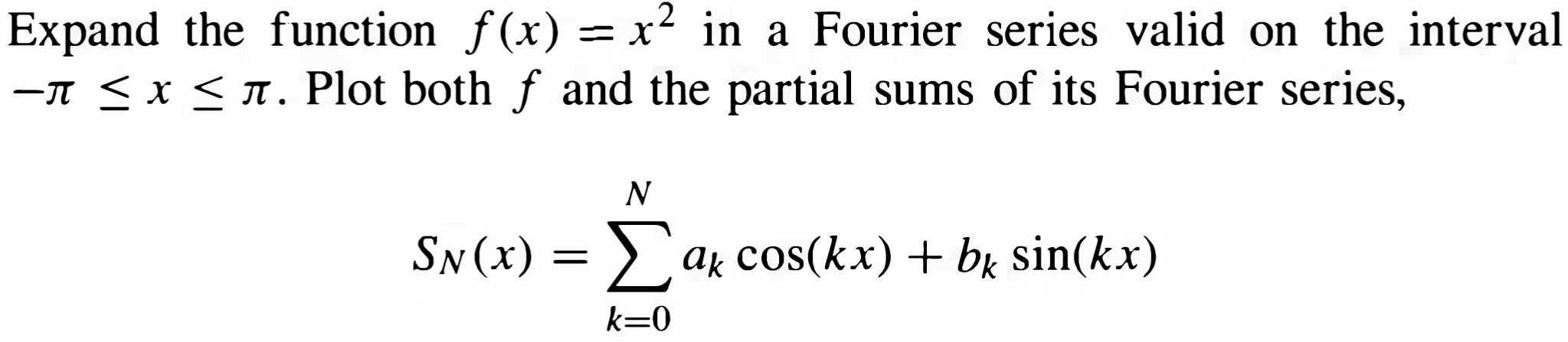Home / Answered Questions / Other / expand-the-function-f-x-x2-in-a-fourier-series-valid-on-the-interval-1-x-1-plot-both-f-and-the-parti-aw568

# (Solved): Expand The Function F(x) = X2 In A Fourier Series Valid On The Interval -1 < X < 1. Plot Both F And ...Expand the function f(x) = x2 in a Fourier series valid on the interval -1 < x < 1. Plot both f and the partial sums of its Fourier series, N. Sn(x) = ax cos(kx) + bk sin(kx) k=0

We have an Answer from Expert